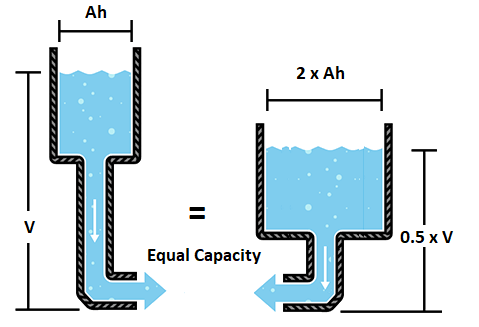# How to Determine the Real Range of an Electric Scooter and its Battery

Before jumping into the Physics of it, you can access our online range calculator here.

Batteries are the lifeblood of any electric vehicle. They are not only their most expensive individual component of a vehicle, but they also define their range and performance. How are they characterised and what do their specifications mean for you, the rider?

Typically batteries are defined by 3 basic specifications, Voltage (V), Amp-hours (Ah) and Watt-hours (Wh).

Let us start by describing Voltage and Amps. Voltage and Amperage are characteristics of electric systems that can easily be understood by using the analogy of water flowing in a pipe. Voltage is akin to water pressure, the more voltage the more ‘pressure’ there is in the current. The Amperage is akin to the flow rate, or the amount of water that is flowing through the pipe. The more Amperage, the more current is ‘flowing’.

The Amps being akin to how much water is flowing, the Amp-hours (Ah) are therefore akin to how much water there is in the tank. 1 Ah is basically the ‘amount of electricity’ equal to 1 Amp of flow for 1 hour.If a battery were simply a water tower, the Voltage is the water pressure (height of the tower) and the Amp-hours is the amount of water in the tank.

Now, how does all this relate to the range of an electric vehicle (electric bike, electric scooter)? The range is directly proportional to the amount of energy stored in the battery. The total energy capacity of a battery is defined in Watt-hours (Wh). Just like Amp-hours, a battery with 1 Wh capacity can provide 1 Watt of power for 1 hour.

You can easily calculate the Watt-hours (Wh) from the Voltage (V) and the Amp-hours (Ah):
Wh=V x Ah

And therefore, the range a battery can provide is directly linked to its Voltage and its Amp-hours. It is a common misconception that a battery’s capacity is measured with Ah. The capacity of a battery is actually measured by Wh which is equal to V x Ah. This means that a 48V battery with 8.8AH capacity (48×8.8 = 422Wh) is actually much larger and will provide longer autonomy than a 24V battery with 12AH (24×12 = 288Wh).To sum up, whenever you want to compare batteries with regards to range, ensure you are not comparing Ah or V. You should be comparing Wh. And the Wh can easily be calculated from V x Ah.

1.Shyam says:
1.Eliott Wertheimer says: